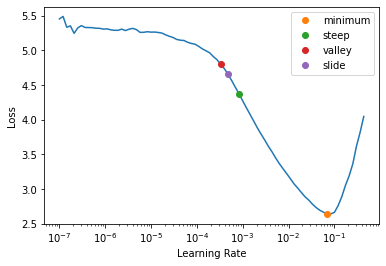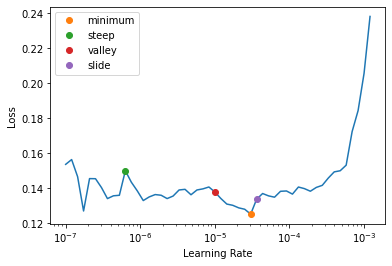# Building a State-of-the-Art Pet Breed Classifier Without Breaking a Sweat

Chapter 5 of Fastbook contains very helpful tips on how to achieve world-class results with deep learning. In this post, we'll use those tips to build a state-of-the-art pet breed classifier.

In particular, we will look at the following techniques:

• Presizing during data augmentation
• Using transfer learning with pre-trained models
• Finding the best learning rate
• Using discriminative learning rates
• Training for the right number of epochs
• Experimenting with deeper architectures

from fastai.vision.all import *

path = untar_data(URLs.PETS)

(path/'images').ls()
(#7393) [Path('/root/.fastai/data/oxford-iiit-pet/images/Russian_Blue_28.jpg'),Path('/root/.fastai/data/oxford-iiit-pet/images/Ragdoll_18.jpg'),Path('/root/.fastai/data/oxford-iiit-pet/images/boxer_175.jpg'),Path('/root/.fastai/data/oxford-iiit-pet/images/german_shorthaired_9.jpg'),Path('/root/.fastai/data/oxford-iiit-pet/images/saint_bernard_28.jpg'),Path('/root/.fastai/data/oxford-iiit-pet/images/staffordshire_bull_terrier_39.jpg'),Path('/root/.fastai/data/oxford-iiit-pet/images/boxer_36.jpg'),Path('/root/.fastai/data/oxford-iiit-pet/images/Bengal_179.jpg'),Path('/root/.fastai/data/oxford-iiit-pet/images/Birman_118.jpg'),Path('/root/.fastai/data/oxford-iiit-pet/images/scottish_terrier_153.jpg')...]

The images folder contains all the images we are interested in. The file name of the images also contains the name of the breed. We can extract these labels from the file name using a regular expression in our data block.

pets = DataBlock(
blocks = (ImageBlock, CategoryBlock),
get_items = get_image_files,
splitter = RandomSplitter(seed=42),
get_y = using_attr(RegexLabeller(r'(.+)_\d+.jpg'), 'name'),
item_tfms=Resize(460),
batch_tfms = aug_transforms(size=224, min_scale=0.75)
)

There are two transforms that have been used in the data block: one for each item, and another for each batch.

First, each image is resized to 460x460. This is much larger than the size that we will feed to our model.

When images are then batched, augmentations are applied on the GPU for the entire batch and in the last step of this augmentation, the images are resized to 224x224.

This approach is known as presizing and it helps greatly reduce the amount of empty zones and degraded data that would otherwise occur if we resize the images directly to 224x224 and perform augmentations on them.

Before we move on, let us check if we have set everything up correctly.

pets.summary(path/'images')
Setting-up type transforms pipelines Collecting items from /root/.fastai/data/oxford-iiit-pet/images Found 7390 items 2 datasets of sizes 5912,1478 Setting up Pipeline: PILBase.create Setting up Pipeline: partial -> Categorize -- {'vocab': None, 'sort': True, 'add_na': False} Building one sample Pipeline: PILBase.create starting from /root/.fastai/data/oxford-iiit-pet/images/english_cocker_spaniel_199.jpg applying PILBase.create gives PILImage mode=RGB size=500x281 Pipeline: partial -> Categorize -- {'vocab': None, 'sort': True, 'add_na': False} starting from /root/.fastai/data/oxford-iiit-pet/images/english_cocker_spaniel_199.jpg applying partial gives english_cocker_spaniel applying Categorize -- {'vocab': None, 'sort': True, 'add_na': False} gives TensorCategory(18) Final sample: (PILImage mode=RGB size=500x281, TensorCategory(18)) Collecting items from /root/.fastai/data/oxford-iiit-pet/images Found 7390 items 2 datasets of sizes 5912,1478 Setting up Pipeline: PILBase.create Setting up Pipeline: partial -> Categorize -- {'vocab': None, 'sort': True, 'add_na': False} Setting up after_item: Pipeline: Resize -- {'size': (460, 460), 'method': 'crop', 'pad_mode': 'reflection', 'resamples': (2, 0), 'p': 1.0} -> ToTensor Setting up before_batch: Pipeline: Setting up after_batch: Pipeline: IntToFloatTensor -- {'div': 255.0, 'div_mask': 1} -> Flip -- {'size': None, 'mode': 'bilinear', 'pad_mode': 'reflection', 'mode_mask': 'nearest', 'align_corners': True, 'p': 0.5} -> RandomResizedCropGPU -- {'size': (224, 224), 'min_scale': 0.75, 'ratio': (1, 1), 'mode': 'bilinear', 'valid_scale': 1.0, 'max_scale': 1.0, 'p': 1.0} -> Brightness -- {'max_lighting': 0.2, 'p': 1.0, 'draw': None, 'batch': False} Building one batch Applying item_tfms to the first sample: Pipeline: Resize -- {'size': (460, 460), 'method': 'crop', 'pad_mode': 'reflection', 'resamples': (2, 0), 'p': 1.0} -> ToTensor starting from (PILImage mode=RGB size=500x281, TensorCategory(18)) applying Resize -- {'size': (460, 460), 'method': 'crop', 'pad_mode': 'reflection', 'resamples': (2, 0), 'p': 1.0} gives (PILImage mode=RGB size=460x460, TensorCategory(18)) applying ToTensor gives (TensorImage of size 3x460x460, TensorCategory(18)) Adding the next 3 samples No before_batch transform to apply Collating items in a batch Applying batch_tfms to the batch built Pipeline: IntToFloatTensor -- {'div': 255.0, 'div_mask': 1} -> Flip -- {'size': None, 'mode': 'bilinear', 'pad_mode': 'reflection', 'mode_mask': 'nearest', 'align_corners': True, 'p': 0.5} -> RandomResizedCropGPU -- {'size': (224, 224), 'min_scale': 0.75, 'ratio': (1, 1), 'mode': 'bilinear', 'valid_scale': 1.0, 'max_scale': 1.0, 'p': 1.0} -> Brightness -- {'max_lighting': 0.2, 'p': 1.0, 'draw': None, 'batch': False} starting from (TensorImage of size 4x3x460x460, TensorCategory([18, 5, 5, 0], device='cuda:0')) applying IntToFloatTensor -- {'div': 255.0, 'div_mask': 1} gives (TensorImage of size 4x3x460x460, TensorCategory([18, 5, 5, 0], device='cuda:0')) applying Flip -- {'size': None, 'mode': 'bilinear', 'pad_mode': 'reflection', 'mode_mask': 'nearest', 'align_corners': True, 'p': 0.5} gives (TensorImage of size 4x3x460x460, TensorCategory([18, 5, 5, 0], device='cuda:0')) applying RandomResizedCropGPU -- {'size': (224, 224), 'min_scale': 0.75, 'ratio': (1, 1), 'mode': 'bilinear', 'valid_scale': 1.0, 'max_scale': 1.0, 'p': 1.0} gives (TensorImage of size 4x3x224x224, TensorCategory([18, 5, 5, 0], device='cuda:0')) applying Brightness -- {'max_lighting': 0.2, 'p': 1.0, 'draw': None, 'batch': False} gives (TensorImage of size 4x3x224x224, TensorCategory([18, 5, 5, 0], device='cuda:0')) 

Looks good! We can now create DataLoaders from this data block.

dls = pets.dataloaders(path/'images')

Instead of training a model from scratch, we use transfer learning. This is where we take a model that is trained for another task or a different dataset, and fine-tune its parameters for the task we are interested in.

Let us start with a resnet50 model trained on the ImageNet dataset.

learn = cnn_learner(dls, resnet50, metrics=error_rate)

Under the hood, the final layer of the network is discarded because the number of outputs it has depends on the task it was trained for - in this case, classification on the ImageNet dataset. A new layer that contains the correct number of outputs required for our task is then attached to the model.

Before we start training this model on our dataset, we need to decide the learning rate to use. The learning rate determines how efficiently a model is trained. Using a small learning rate requires the model to be trained for more epochs. On the other hand, using a large learning rate may prevent the loss from settling at a minimum. With a large enough learning rate, the loss may even begin to diverge.

In 2015, Leslie Smith published a paper where he came up with the idea of a learning rate finder - a method to find the ideal learning rate.

The process works as follows:

1. Set the learning rate to an extremely small value.
2. Increase the learning rate by a certain percentage for each mini-batch (e.g. double the learning rate for each mini-batch) and record the loss value.
3. Keep repeating this process until the loss gets worse instead of getting better.

Fastai has this procedure available in the lr_find method.


lr_min, lr_steep, lr_valley, lr_slide = learn.lr_find(suggest_funcs=(minimum, steep, valley, slide))lr_min, lr_steep, lr_valley, lr_slide
(0.006918309628963471,
0.0008317637839354575,
0.0003311311302240938,
0.0004786300996784121)

Using the learning rate finder, we've asked fastai to suggest 4 different learning rates:

1. minimum - one-tenth the value of the learning rate where the loss is minimum.
2. steep - learning rate at which the slope of the loss is steepest.
3. valley - the steepest slope roughly 2/3 through the longest valley.
4. slide - learning rate following an interval slide rule.

More details about these methods can be found in the fastai documentation.

Let us train the new head for a few epochs using the learning rate at the steepest slope

learn.fit_one_cycle(5, lr_steep)
epoch train_loss valid_loss error_rate time
0 1.317904 0.266430 0.083221 01:16
1 0.525201 0.243140 0.070365 01:15
2 0.324229 0.210824 0.059540 01:16
3 0.211695 0.175427 0.049391 01:16
4 0.181848 0.177565 0.048714 01:17

The newly attached "head" of the model has completely random weights. If we try to train the entire model then we will destroy the perfectly well-trained parameters in the earlier layers of the model. To prevent this, we first trained only the parameters of the newly added layer with all earlier layers frozen.

Now we are ready to unfreeze the pre-trained layers and train all the layers in the model.

learn.unfreeze()

The earlier layers of a model may not need as high a learning rate as the later layers, especially the newly added final layer. Using a high learning rate for the earlier layers may still destroy the parameters that have been learning by training for many epochs on enormous datasets.

A Python slice object can be passed anywhere a learning rate is expected in fastai. The first value will be the learning rate of the earliest layer, the second will be the learning rate of the final layer. The layers in between will have learning rates that are multiplicatively equidistant throughout the range.

But first, since we've unfrozen layers and there are more parameters to train, let's run the learning rate finder again to find the best learning rate.

lr_min, lr_steep, lr_valley, lr_slide = learn.lr_find(suggest_funcs=(minimum, steep, valley, slide))lr_min, lr_steep, lr_valley, lr_slide
(3.0199516913853586e-06,
6.309573450380412e-07,
9.999999747378752e-06,
3.630780702224001e-05)

The graph looks much different from the first time we ran the test because the head of our model has been fine-tuned already.

We can now use lr_steep for the earliest layer and lr_valley for the last layer.

In general, you should use a learning rate before the sharp increase in the loss.

learn.fit_one_cycle(10, lr_max=slice(lr_steep, lr_valley))
epoch train_loss valid_loss error_rate time
0 0.149643 0.175833 0.047361 01:31
1 0.162749 0.176478 0.049391 01:30
2 0.152322 0.178343 0.048714 01:31
3 0.143987 0.170578 0.046008 01:32
4 0.138569 0.167886 0.043978 01:31
5 0.142414 0.171065 0.046008 01:31
6 0.128563 0.166929 0.044655 01:31
7 0.130237 0.168690 0.043302 01:32
8 0.128327 0.169738 0.043978 01:31
9 0.122282 0.168022 0.045332 01:31

The number of epochs I have trained for was not a random choice. I actually trained the model for many more epochs and saw when the error_rate started increasing. Then I retrained the model for just those many epochs.

In general, you need to stop training when the metrics you are interested in started getting worse, and not the loss function. The loss function is there just to allow the optimizer to differentiate and update the parameters of the model. It's the metrics that we really care about.

Also, the pre-trained model I selected was resnet50. You can also experiment with shallower or deeper models. Models with more layers can capture more complex relationships in data. However, they can also memorize the data more easily and start overfitting.

Further, when the number of layers in a model is increased, the batch size may need to be reduced to keep the GPU from running out of memory.

Deeper models also take much longer to train. However, training can be sped up using half-precision floating point numbers.

## Conclusion

In this short post, we achieved state-of-the-art classification accuracy without breaking a sweat! According to the Papers with Code page of this dataset, our model would be in the top 5 with an accuracy of 95.46%! (Place 4 to be precise, at the time of writing this post).

The concepts covered in chapter 5 of fastbook and in this post can be reused for other datasets and tasks as well.

To summarize,

• Use presizing to reduce the amount of data degradation during augmentation of image datasets